Courses
Courses for Kids
Free study material
Free LIVE classes
MoreLIVE
Join Vedantu’s FREE Mastercalss

## NCERT Class 5 Maths Chapter 8 Mapping Your Way: Revision Notes

Class 5 Maths Chapter 8 Mapping Your Way is a crucial chapter of this syllabus that teaches the different aspects and elements of maps that students should know. Apart from the map details, they will also learn how to use a map and use the mathematical concepts taught in this chapter to focus on building their concepts. This is where the notes designed by the subject experts of Vedantu can be used perfectly.

Class 5 Maths Chapter 8 is a unique part of the curriculum wherein students will be taught how to read maps and how to find directions. They will also learn to interpret time and distance by using a map scale. Download and refer to the Mapping Your Way revision notes to understand the concepts covered in this chapter.

Learn how to interpret the features of a particular map with the help of these revision notes. In this chapter, you will also learn how to read the directions to be followed on maps. Understand the objective of the Mapping Your Way summary and find out how to use the map scale to determine distances.

Last updated date: 18th Sep 2023
Total views: 142.8k
Views today: 3.42k

## Directions:

• Direction is a way or path in which things move.

• There are four cardinal directions.

• For example, you are facing toward the East.

 Cardinal directions: The directions that are defined by taking the Sun as a reference.

## How to Find Directions?

We need a device called Compass, to find the Cardinal directions.

• A compass is a device that helps to find directions.

• It consists of a needle made of a magnet that always turns in the North direction.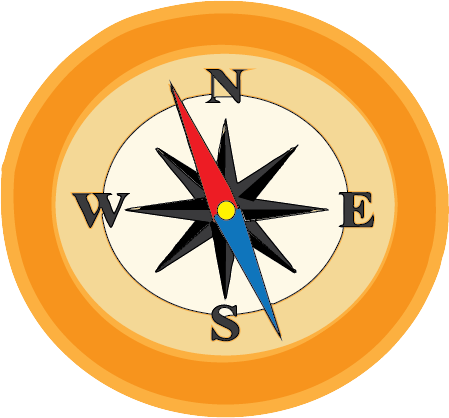Compass

• In the Compass image, ‘N’ stands for North, ‘S’ for South, ‘E’ for East and ‘W’ for West.

• We know that the Sun rises in the East and sets in the West. Hence they are in opposite directions. Whereas North and South are opposite to each other.

• From the Compass image, we can say that North is always in the right direction to the East and South is to the left.

Analyze the given image and answer the following questions.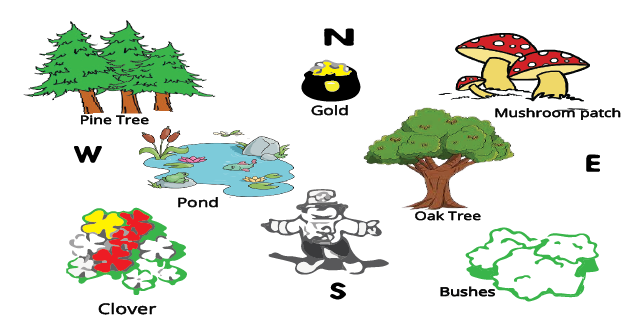Directions of object

Solved example 1: The pond is in which direction of the oak tree?

Ans: The pond is located in the West direction.

Solved example 2: The gold pot is placed in which direction?

Ans: Gold pot is placed in the North direction.

Solved example 3: The direction that is opposite the pond is …

Ans: Pond is in the West direction hence the direction opposite to West is East.

## Map Scale

• Since it is difficult to add the exact distance on the map, we use a term called Scale.

• In the image, it mentions that “1 cm = 2 km”, which means ‘1 cm’ on the map is equal to ‘2 km’ on the Earth.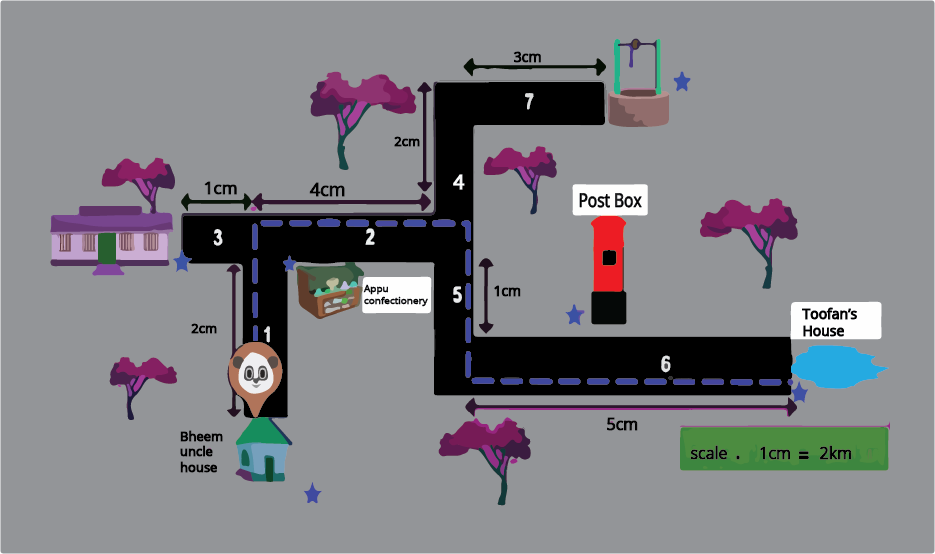Map Scale

Now let us answer some questions with reference to the above image,

Solved example 1: What is the path taken by Panda?

Ans: The path chosen by Panda on the map is along 1, 2, 5, and 6. Panda starts at Bheem Uncle's house, then goes along with Appu Confectionery, then crosses Post Box and reaches Toofan’s house.

Solved example 2: Find the distance from Bheem's uncle's house to Toofan's house. (Hint: Distance is the length between two points.)

Ans: To find the distance, let us first add the total scale from Bheem's uncle's house to Toofan’s House. Follow paths 1, 2, 5, and 6 on the map.

2 cm + 4 cm + 1 cm + 5 cm

From the scale it is given that 1 cm = 2 km. Hence we should replace the 1 cm value with 2 km.

Hence we can write the scale values as

2 cm = 1 cm + 1 cm = 2 km + 2 km = 4 km

4 cm = 1 cm + 1 cm + 1 cm + 1 cm = 2 km + 2 km + 2 km + 2 km = 8 km

1 cm = 2 km

5 cm = 1 cm + 1 cm + 1 cm + 1 cm + 1 cm = 2 km + 2 km + 2 km + 2 km + 2 km = 10 km

Now let us add all the values,

4 km + 8 km + 2 km + 10 km

24 km

Solved example 3: From the map which is the longest road?

Ans: By looking at the map we can say that the largest scale number in the path chosen by Panda is the longest road. Hence 5 cm is the largest number, number 6 is the longest road.

## Uses of Scale and Directions in Map

• The scale is used in the maps to find the original distance between two places.

• Whereas the directions help us to locate the place on a map.

Let’s solve some more examples by using scale.

Solved example 1: In a map, the scale is 1 cm of map = 5 km on Earth, then fill in the blank.

13 cm = ……….. km.

Ans: Follow the same method of the solved example given above,

1 cm = 5 km

13 cm = ?   km

13 * 5 = 65 km

Solved example 2: From the image, the largest distance between A and B is in Map - 1 or Map - 2.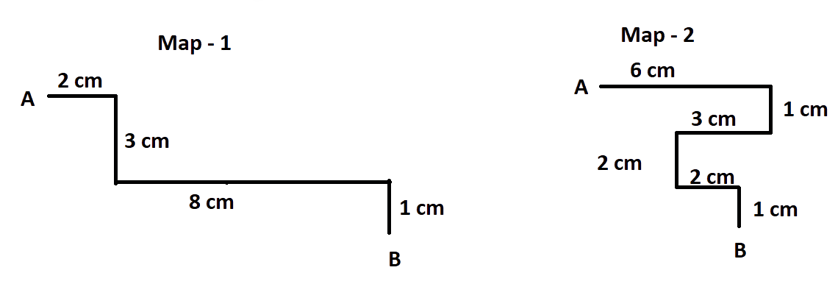Distance between A and B

Ans: Let us first calculate the distance between A and B in Map - 1 and 2

Distance in Map - 1 = 2 cm + 3 cm + 8 cm + 1 cm = 14 cm

Distance in Map - 2 = 6 cm + 1 cm + 3 cm + 2 cm + 2 cm + 1 cm = 15 cm

Hence from both the distances, we can say that distance in Map - 2 is the largest.

## Practise Questions

Q1. Answer the following questions, with reference to the map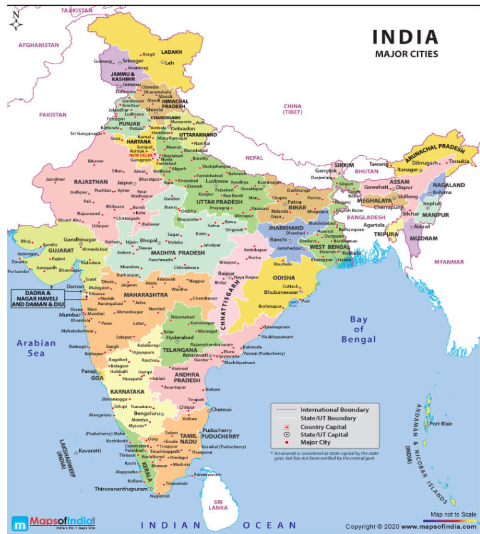India Map

1. According to the map, the place that is located in the North is ………..

2. What is the direction of the Arabian Sea?

3. The Bay of Bengal is located in the East direction. True / False

Ans: We can solve these questions by placing the compass given in the image exactly placing it in the centre of the map.

1. New Delhi

2. West

3. True

Q2: The sun rises in the east. Hence north and south directions are in right and left of the east respectively. True / False

Ans: True

## Importance of CBSE Class 5 Maths Chapter 8 Mapping Your Way

• This chapter will teach you how to check a map and determine the distance using map scales. Maps are drawn following a scale. This scale can be used to interpret distance when the maps are accurate.

• Learn how to check directions to reach a destination. You will also learn how roads, boundaries, and borders are depicted on a map. The chapter will teach you how to read maps and find distances between towns.

• This chapter will help you depict the meaning of map scales and figure out how to solve the related problems given in the exercises. In fact, you will understand how to answer questions related to making it bigger and making it smaller by using map scales on your own.

• Understanding a map will become easier when you learn the concepts in this chapter. Also, you will learn to match the map and the photo and extract proper information from the illustrations.

## Benefits of Mapping Your Way Class 5 Worksheets and Revision Notes

Our subject experts have designed the revision notes based on the CBSE guidelines to ensure that the objectives of this chapter are preserved. Here is how you can benefit from the free PDF of revision notes and worksheets with answers.

• The explanation of the concepts has been given in a simple way. You will understand the fundamental principles of recognizing map features quickly. You will comprehend the meaning of questions and answer them accurately.

• As this is a new conceptual chapter introduced for the first time, focus on solving the exercise questions first. Solve and practice the Mapping Your Way Class 5 worksheets with answers. Compare your answers with the given solutions to check your answers. In this way, you will be able to identify if there are any errors in your solutions and prepare the chapter effectively.

## Importance of NCERT Class 5 Maths Chapter 8 Mapping Your Way Revision Notes

The preparation of a chapter depends on the study material a student has organised. In the same context, NCERT Class 5 Maths Chapter 8 Mapping Your Way Revision Notes will become an integral part of the study material a student needs to understand the context and mathematical concepts of this chapter.

These notes focus on the CBSE Class 5 Maths syllabus and cover all the topics of this chapter skilfully. Students will find out the simpler version of the concepts and topics added to this chapter in these notes to complete preparing it well. They will find the highest convenience to use these notes and revise before an exam.

The easier version of the notes can be used to study this chapter well and recall what they have studied precisely during an exam. These organised notes will enable students to focus on how to use the concepts well to formulate the right answers to the fundamental questions.

CBSE Class 5 Maths Chapter 8 Mapping Your Way Notes can be accessed online or can be downloaded in PDF format for more convenience. You can access these notes offline or online according to your academic requirements and take your preparation to the next level.

Get the free PDF version of these notes and complete your study material for this chapter. Focus on the concepts and principles only by using these notes during revision. Complete revising this chapter efficiently and quickly and recall what you have studied by using the simpler version of these notes. Develop your concepts accordingly and learn how to score more in the exams by using these notes.

1.  How can I answer questions related to waiting for the parade?

Simply focus on the map and read the scale. Find the position of the parade on the route. Check the distance on the map and use the scale to determine the actual distance. Interpret it with time to find out when the parade will reach your point.

2.  What is the best way to measure distance on a map?

If the roads are straighter than use a scale. You can use the map scale to interpret the distance. If the roads are winding in nature, you can use a thread, and mark the starting point, ending point, and the turns. Measure the length between the markings on the thread to get the required distance on map.

3.  Where can I get good NCERT Solutions for Class 5 Maths Chapter 8?

You can get NCERT Solutions for Class 5 Maths Chapter 8 prepared by subject experts on Vedantu mobile app and official website. These solutions are available free of cost. Refer to thNCERT Solutions, follow the steps to practice, and answer the questions accurately.

4. How can I resolve doubts with revision notes for this chapter?

Resolve doubts related to the concepts taught in this chapter anytime by using the simpler version of the topics and concepts. Find out the correct answers to all your queries from these notes.

5. Where can I find the simpler explanation of the concepts related to Class 5 Maths Chapter 8 Mapping Your Way?

Focus on the methods of explaining the topics and mathematical principles of this chapter. Learn how to use them to solve exercise questions.